Anúncio

# Magellan-Spark as a Geospatial Analytics Engine by Ram Sriharsha

24 de Feb de 2016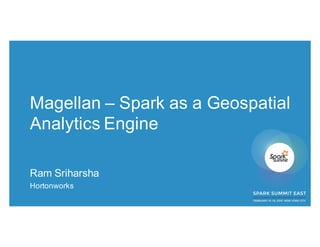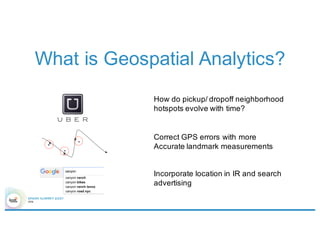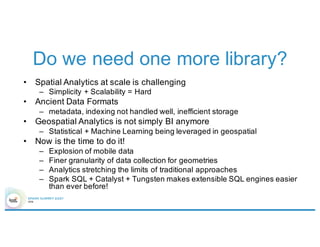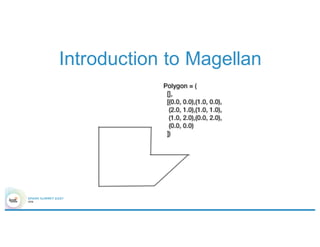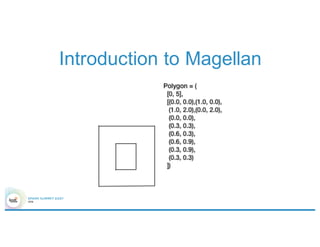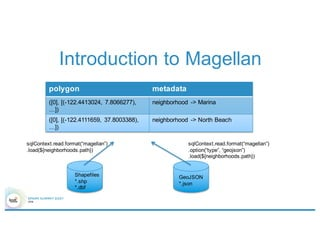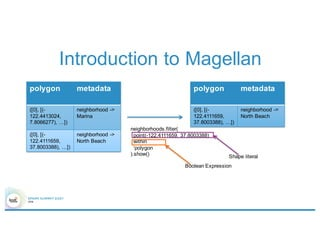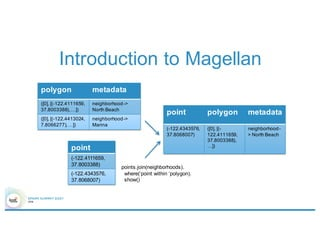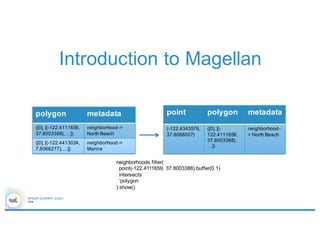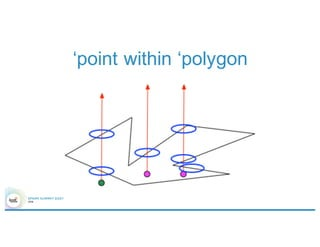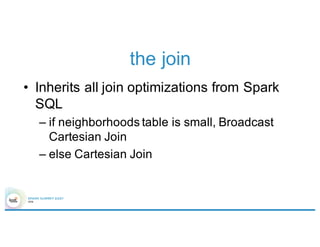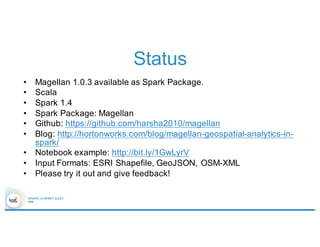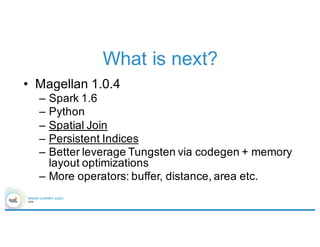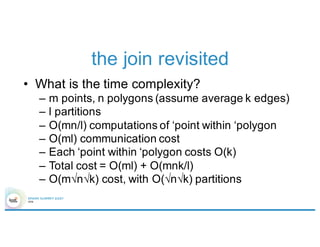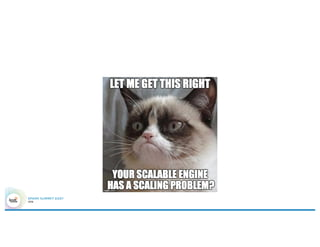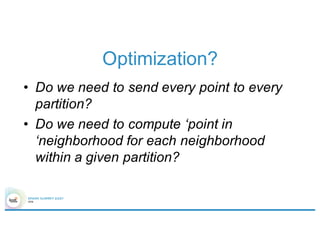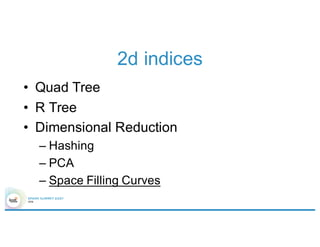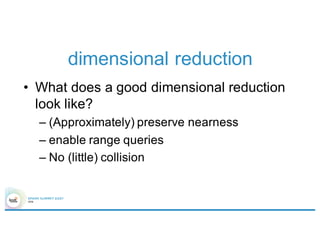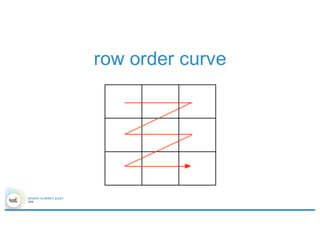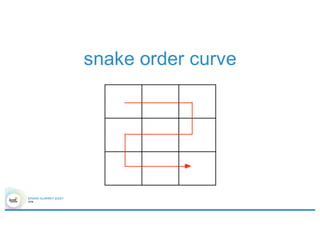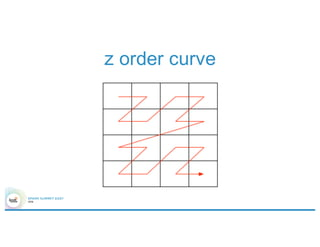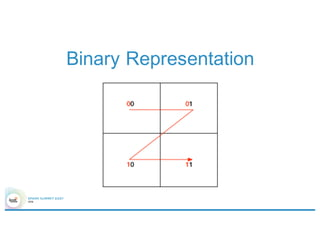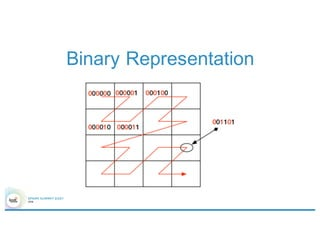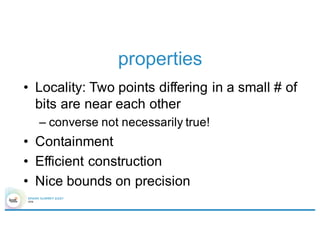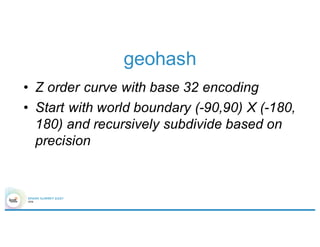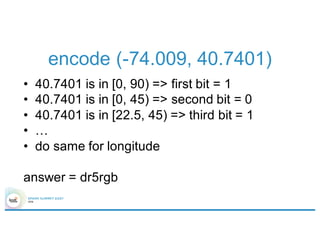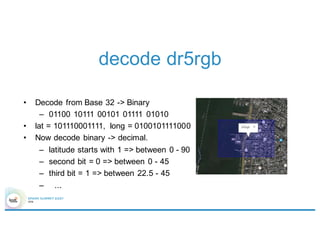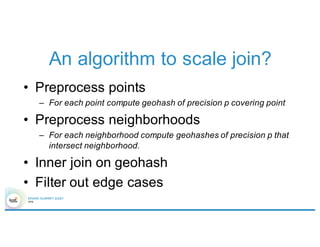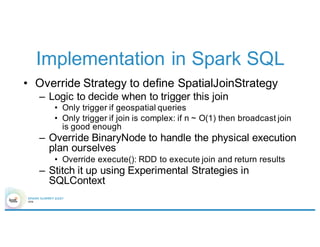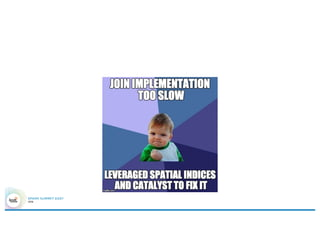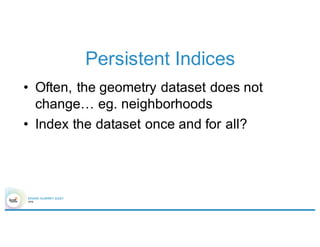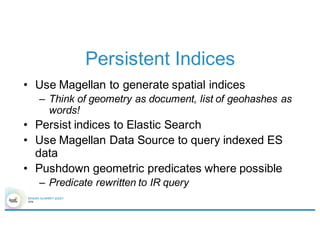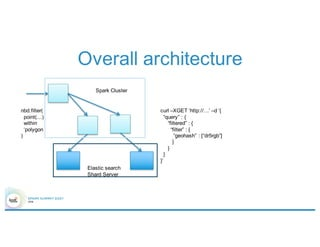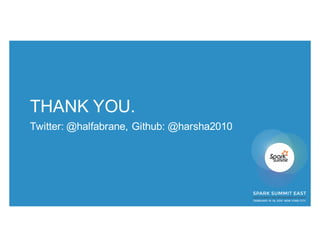1 de 36
Anúncio

### Magellan-Spark as a Geospatial Analytics Engine by Ram Sriharsha

1. Magellan – Spark as a Geospatial Analytics Engine Ram Sriharsha Hortonworks
2. Who Am I? • Apache Spark PMC Member • Hortonworks Architect, Spark + Data Science • Prior to HWX, Principal Research Scientist @ Yahoo Labs (Large Scale Machine Learning) – Login Risk Detection, Sponsored Search Click Prediction, Advertising Effectiveness Models, Online Learning, …
3. What is Geospatial Analytics? How do pickup/ dropoff neighborhood hotspots evolve with time? Correct GPS errors with more Accurate landmark measurements Incorporate location in IR and search advertising
4. Do we need one more library? • Spatial Analytics at scale is challenging – Simplicity + Scalability = Hard • Ancient Data Formats – metadata, indexing not handled well, inefficient storage • Geospatial Analytics is not simply BI anymore – Statistical + Machine Learning being leveraged in geospatial • Now is the time to do it! – Explosion of mobile data – Finer granularity of data collection for geometries – Analytics stretching the limits of traditional approaches – Spark SQL + Catalyst + Tungsten makes extensible SQL engines easier than ever before!
5. Introduction to Magellan
6. Introduction to Magellan
7. Introduction to Magellan Shapefiles *.shp *.dbf sqlContext.read.format(“magellan”) .load(\${neighborhoods.path}) GeoJSON *.json sqlContext.read.format(“magellan”) .option(“type”, “geojson”) .load(\${neighborhoods.path}) polygon metadata (, [(-122.4413024, 7.8066277), …]) neighborhood -> Marina (, [(-122.4111659, 37.8003388), …]) neighborhood -> North Beach
8. Introduction to Magellan polygon metadata (, [(- 122.4413024, 7.8066277), …]) neighborhood -> Marina (, [(- 122.4111659, 37.8003388), …]) neighborhood -> North Beach neighborhoods.filter( point(-122.4111659, 37.8003388) within ‘polygon ).show() polygon metadata (, [(- 122.4111659, 37.8003388), …]) neighborhood -> North Beach Shape literal Boolean Expression
9. Introduction to Magellan polygon metadata (, [(-122.4111659, 37.8003388),…]) neighborhood-> North Beach (, [(-122.4413024, 7.8066277),…]) neighborhood-> Marina point (-122.4111659, 37.8003388) (-122.4343576, 37.8068007) points.join(neighborhoods). where(‘point within ‘polygon). show() point polygon metadata (-122.4343576, 37.8068007) (, [(- 122.4111659, 37.8003388), …]) neighborhood- > North Beach
10. Introduction to Magellan polygon metadata (, [(-122.4111659, 37.8003388),…]) neighborhood-> North Beach (, [(-122.4413024, 7.8066277),…]) neighborhood-> Marina neighborhoods.filter( point(-122.4111659, 37.8003388).buffer(0.1) intersects ‘polygon ).show() point polygon metadata (-122.4343576, 37.8068007) (, [(- 122.4111659, 37.8003388), …]) neighborhood- > North Beach
11. ‘point within ‘polygon
12. the join • Inherits all join optimizations from Spark SQL – if neighborhoods table is small, Broadcast Cartesian Join – else Cartesian Join
13. Status • Magellan 1.0.3 available as Spark Package. • Scala • Spark 1.4 • Spark Package: Magellan • Github: https://github.com/harsha2010/magellan • Blog: http://hortonworks.com/blog/magellan-geospatial-analytics-in- spark/ • Notebook example: http://bit.ly/1GwLyrV • Input Formats: ESRI Shapefile, GeoJSON, OSM-XML • Please try it out and give feedback!
14. What is next? • Magellan 1.0.4 – Spark 1.6 – Python – Spatial Join – Persistent Indices – Better leverage Tungsten via codegen + memory layout optimizations – More operators: buffer, distance, area etc.
15. the join revisited • What is the time complexity? – m points, n polygons (assume average k edges) – l partitions – O(mn/l) computations of ‘point within ‘polygon – O(ml) communication cost – Each ‘point within ‘polygon costs O(k) – Total cost = O(ml) + O(mnk/l) – O(m√n√k) cost, with O(√n√k) partitions
16. Optimization? • Do we need to send every point to every partition? • Do we need to compute ‘point in ‘neighborhood for each neighborhood within a given partition?
17. 2d indices • Quad Tree • R Tree • Dimensional Reduction – Hashing – PCA – Space Filling Curves
18. dimensional reduction • What does a good dimensional reduction look like? – (Approximately) preserve nearness – enable range queries – No (little) collision
19. row order curve
20. snake order curve
21. z order curve
22. Binary Representation
23. Binary Representation
24. properties • Locality: Two points differing in a small # of bits are near each other – converse not necessarily true! • Containment • Efficient construction • Nice bounds on precision
25. geohash • Z order curve with base 32 encoding • Start with world boundary (-90,90) X (-180, 180) and recursively subdivide based on precision
26. encode (-74.009, 40.7401) • 40.7401 is in [0, 90) => first bit = 1 • 40.7401 is in [0, 45) => second bit = 0 • 40.7401 is in [22.5, 45) => third bit = 1 • … • do same for longitude answer = dr5rgb
27. decode dr5rgb • Decode from Base 32 -> Binary – 01100 10111 00101 01111 01010 • lat = 101110001111, long = 0100101111000 • Now decode binary -> decimal. – latitude starts with 1 => between 0 - 90 – second bit = 0 => between 0 - 45 – third bit = 1 => between 22.5 - 45 – ...
28. An algorithm to scale join? • Preprocess points – For each point compute geohash of precision p covering point • Preprocess neighborhoods – For each neighborhood compute geohashes of precision p that intersect neighborhood. • Inner join on geohash • Filter out edge cases
29. Implementation in Spark SQL • Override Strategy to define SpatialJoinStrategy – Logic to decide when to trigger this join • Only trigger if geospatial queries • Only trigger if join is complex: if n ~ O(1) then broadcast join is good enough – Override BinaryNode to handle the physical execution plan ourselves • Override execute(): RDD to execute join and return results – Stitch it up using Experimental Strategies in SQLContext
30. Persistent Indices • Often, the geometry dataset does not change… eg. neighborhoods • Index the dataset once and for all?
31. Persistent Indices • Use Magellan to generate spatial indices – Think of geometry as document, list of geohashes as words! • Persist indices to Elastic Search • Use Magellan Data Source to query indexed ES data • Pushdown geometric predicates where possible – Predicate rewritten to IR query
32. Overall architecture Elastic search Shard Server Spark Cluster nbd.filter( point(…) within ‘polygon ) curl –XGET ‘http://…’ –d ‘{ “query” : { “filtered” : { “filter” : { “geohash” : [“dr5rgb”] } } } }’
33. THANK YOU. Twitter: @halfabrane, Github: @harsha2010
Anúncio